﻿ NLO Application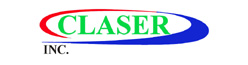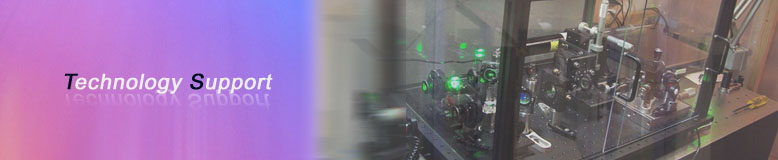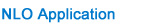NLO crystals Application

Frequency Conversion

The frequency conversion processes include frequency doubling (which is a special case of sum frequency generation), sum frequency generation (SFG), differential-frequency generation (DFG) and optical parametric generation (OPG) which are demonstrated in the following equations:

Sum Frequenby Generation (SFG):

ω1+ω2=ω3

(or 1/λ1+1/λ2=1/λ3 in wavelength)

It combines two low energy (or low frequency) photons into a high energy photon. For example:

1064nm+1064nm→532nm

Differential-Frequency Generation (DFG):

ω1-ω2=ω3

(or 1/λ1+1/λ2=1/λ3 in wavelength)

It combines two high energy photons into a low energy photon. For example:

532nm-810nm→1550nm

Optocal Parametric Generatiom (OPG):

ωp-ωs=ωi

(or 1/λp=1/λs+1/λi in wavelength)

It splits one high energy photon into two low energy photons.

Frequency Doubling

Frequency Doubling or Second Harmonic Generation (SHG) is a special case of sum frequency generation if the two input wavelengths are the same.The simplest scheme for frequency doubling is extracavity doubling.The laser passes through the nonlinear crystal only once as shown in the Fig.3. However,if the power density of laser is low,focused beam ,intracavity doubling and external resonant cavity are normally used to increase the power density on the crystals,for example,for doubling of cw Nd:YAG laser and Argon lon lasers.

Sum Frequency Generation

Frequency Tripling or Third Harmonic Generation(THG)is an example of Sum Frequency Generation where,for THG of Nd:YAG laser, w1=1064nm, w2=532nm and THG of a Ti:Sapphire laser in BBO crystal, it can generate wavelength as short as 193 nm.

Optical Parametric Oscillation

Optical Parametric Generation (OPG) is an inverse process of sum Frequency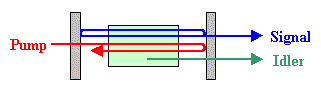Generation. It splits one high-frequency photon (pumping wavelength, lp) into two low-frequency photons (signal, ls, and idler wavelength, li). If two mirrors are added to from a cavity as shown in fig. 7, an Optical Parametric Oscillator (OPO) is established. For a fixed pump wavelength, an infinite number of sigal and idler wavelengths can be generated by tilting a crystal. Therefore, OPO is an excellent source for generating wide tunable range coherent radiation. BBO, KTP, LBO and LINbO3 are good crystal for OPO and Optical Parametic Amplifier (OPA)Applications.

Phase-matching

IN order to obtain high conversion efficiency, the phase vectors of input beams and generated beams have to be matched: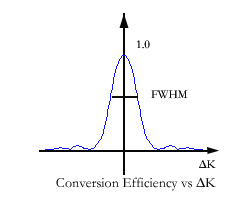ΔK=k3-k2-k1=2p(n3/l3-n2/l2-n1/l1)=0 (For sum frequency generation)

Where:ΔK is phase mismatching, ki is phase vector at li and ni is refractive index atli.

In low power case, the relationship between conversion efficiency and phase mismatching is:

h∞(SIN(ΔKL)/ΔKL)^2

It is clear that the conversion efficiency will drop dramatically if ΔK increases. The phase-matching can be obtained by angle tilting, temperature tuning or other methods. The angle tilting is mostly used to obtain phase-matching as shown. If the angle between optical axis and beam propagation (q) is not equal to 90 deg. or 0 deg., we call it Critical phase-matching (CPM). Otherwise, 90deg. non-critical phase-matching (NCPM) is for q=90deg. and 0 deg. NCPM is for q=0deg.

Two types of phase-matching are classsified in consideration of polarization of lasers. If the polarizations of two input beams (for sum frequency) are parallel to each other., it is type I phase-matching. If the polarizations are perpendicular to each other, it is called type II phase-matching.

Conversion Efficiency

How to select a NLO crystal for a frequency convension process with a certain laser? The most important thing is to obtain a high conversion efficiency. The conversion effciency has the following relationship with effective nonlinear coefficient (Deff), crystal length(L), input power density (P) and phase mismatching (ΔK):

h∞PL^2(DeffSIN(ΔKL)/ΔKL)^2

IN general, higher power density, longer crystal length, larger nonlinear coefficients and smaller phase mismatching will result higher conversion efficiency. However, there is always some limitation coming from nonlinear crystals and lasers. For example, the Deff is determined by the nonlinear crystal itself and the input power density has to be lower than the damage threshold of crystal. Therefore, it is important to select a right crystal for your applications. In the following Table, we list the laser and crystal parameters for selecting right crystals.

 Laser Parameters Crystal Parameters NLO Process Phase-Matching Type and Angle Power or Energy Damage Threshold, Deff Divergence Acceptance Bandwidth Spectral Acceptance Beam Size Crystal Size, Walk-off Angle Pulse Width Group Velocity Mismatching Repetition Rate Damage Threshold Environment Temperature Acceptance, Moisture

Crystal Acceptance

If a laser light propagates in the direction with angle Δq to phase matching direction, the conversion efficiency will reduce dramatically. We define the acceptance angle (Δq) as full angle at half maximum (FAHM), where q=0 deg. is phase-matching direction. For example, the acceptance angle of BBO for type I frequecny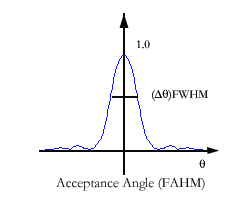doubling of Nd:YAG at 1064nm is about 1mrad-cm. therefore, if a Nd:YAG laser has beam dibergence of 3mrad for frequency-doubling, over half of the input power is useless. In this case, LBO may be better because of its larger acceptance angle, about 8 mrad-cm. For NCMP, the acceptance angle is normally much bigger than that for CPM, for example, 52 mrad-cm(1/2) for type I NCPM LBO.

In addtion, you have to consider the Spectral acceptance (Δl) of crystal and the spectral bandwidth of your laser; crystal temperature acceptance (ΔT)and the temperature change of environment.

Walk-Off

Due to the birefringence of NLO crystals, the extraordinary wave (ne)will experience Poynting vector walk-off as shown. If the beam size of input beam will be separated at walk-offangle(ρ) in the crystal and it will cause low conversion efficiency. Therefore, for focused beam or intracavity doubling, the walk-off is a main limitation to high conversion efficiency.

Group Velocity Mismatching

For NLO processes of ultrafast lasers such as Ti:Sapphire and Dye lasers with femtosecond (fs) pulse width, the main limitation to conversion efficiency is group velocity mismatching(GVM). The GVM is caused by group velocity dispersion of NLO crystal. For frequency doubling a Ti:sapphire laser at 800nm, for example, the inverse group velocities (1/VG)of BBO are respectively 1/VG=56.09ps/cm at 800nm and 1/VG=58.01ps/cm at 400nm and GVM=1.92ps/cm. That means an 1mm long BBO crystal will make 192fs separation between the pulses at two wavelengths. Therefore, for an 100fs Ti:sapphire laser, we normally recommend a 0.5mm long BBO crystal (with 96 fs separation) in order to obtain high effciency without dramatic pulse broadening.

How to Handle A NLO Crystal

Keep crystal clean

When you receive the NLO crystals, plese look at the polished or coated crystal surface first. If the surface are contaminated, please blow the surface with air ball. If there is still pollution on the crystal surfaces, please clean the surfaces with cleaning liquid and soft silk. For BBO crystal, the mixing liqiud of 50% high purity alcohol and 50% high purity ether is recommended as cleaning liquid. Please note that the contaminated surfaces are very easy to be damaged.

Angle Tilting

In order to obtain maximum conversion efficiency, angle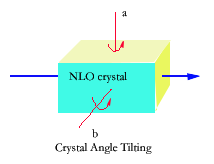tilting is normally used to reach phase-matching direction. There are two axis for tilting crystal angles as show. Becausethe NLO crystals are normally cut in principal crystal plane, conversion efficiency is not sensitive to the angle tilting around b-axis. Customers have to pay attention when rotating the crystal around a-axis. A crystal mount with angle accuracy of about 5 arc second is recommended.

Optimum Crystal Size and Cut

When ordering a nonlinear optical crystal, crystal orientationand size have to be known. The orientation is solely determined by the nonlinear optical process. The crystal size is divided into three dimensions noted as WxHXLmm3. The careful design of crystal size is important because the conversion effciency has direct relation to crystal length.

To select the optimum crystal height(H), the laser beam diameter upon the crystal should be taken into accout. The optimum crystal height should slightly(1-2mm) larger than the laser beam diameter upon the crystal.Both of laser beam Diameter upon NLO crystal and tunable wavelength range have to be considered when designing the optimum crystal width(W). The crystal length is decided by the application. The different crystals and different application will be required the different length's crystals.